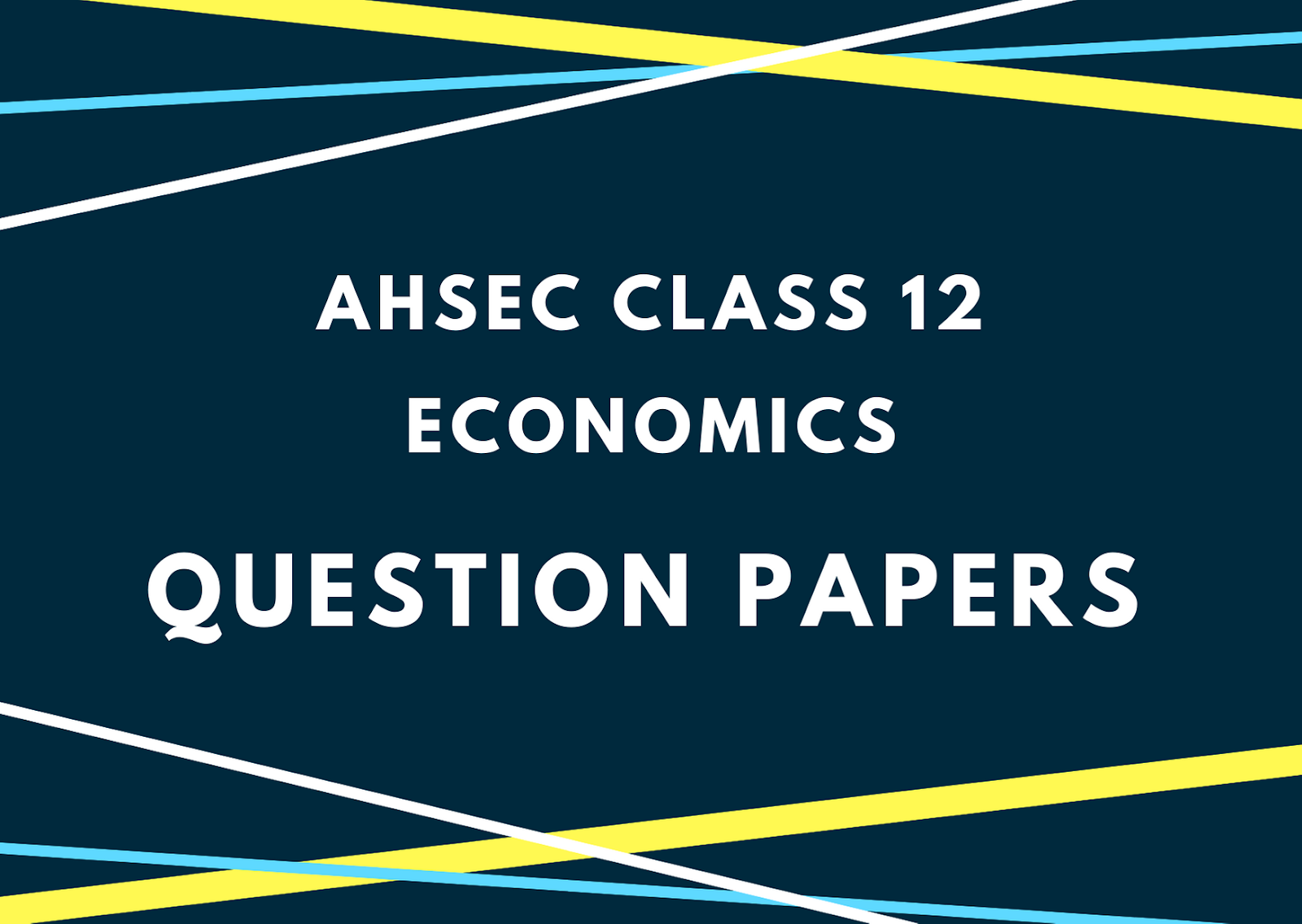# AHSEC Class 12 Economics Question Paper 2014## AHSEC Class 12 Economics Question Papers 2014ACCOUNTANCYFull Marks: 100Pass Marks: 30Time: Three HoursFull Marks: 100 Time: 3 hour
1. (a) What is market economy? 1
(b) Fill in the blank: 1
Opportunity cost is also called the ____ cost.
(c) Define utility. 1
(d) What is marginal revenue? 1
(e) Draw a very short-run supply curve for perishable commodities. 1
(f) Give an example of fixed cost. 1
2. State two central problems of an economy. 2
3. Why is an Isoquants negatively sloped? 2
4. State any two factors affecting the supply of a commodity. 2
5. Distinguish between short-run and long-run. 2
6. State two reasons behind the working of the law of diminishing marginal product. 2
7. Write down the concept of normal profit. 2
8. What is price elasticity of demand? Mention any three important factors determining price elasticity of demand? 1+3=4

9. Suppose, when the price of a commodity is Rs. 10, the quantity supplied is 20 units. As price increases to Rs. 15, the quantity supplied increases to 30 units. Calculate the elasticity of supply. 4
10. The total cost (TC) schedule of a production unit is given below. Find out the average cost (AC) and marginal cost (MC) schedules for the production unit. 2+2=4
 Q TC 1 2 3 4 5 6 7 30 55 75 90 110 140 180

11. Show the relationship between average cost (AC) and marginal cost (MC) with the help of a diagram. 4
12. Write down four characteristics of a perfectly competitive market. 4
13. State and explain the law of demand with the help of a diagram. 6
Or
Explain the concept of short-run equilibrium of a monopoly market assuming zero cost.
14. “In perfect competition, AR = MR but in monopoly AR > Mr.” – Explain. 6
Or
The demand and supply function of a firm under perfectly competitive market are given below:15. (a) In which type of money, the face value is higher than the intrinsic value? 1
(b) Define final goods. 1

(c) What is bank rate? 1
(d) What type of budget should the government prepare in times of inflation? 1
(e) State one example of non-tax revenue. 1
(f) What is invisible trade? 1
16. Distinguish between gross investment and net investment. 2
17. What is personal disposable income? 2
18. The marginal propensity to consume (MPC) of an economy is 0.9 and suppose, an additional sum of Rs. 500 crores is invested in it. How much new income will be generated in the economy? 2
19. What is marginal propensity to save? 2
20. Mention two main accounts of balance of payments. 2
21. What does the revenue account of a government budget contain? 2
22. Distinguish between consumer goods and capital goods. 4
23. The autonomous consumption of an individual is Rs. 500 and his personal disposable income is Rs. 5000. If his marginal propensity to consume is 0.8, find out the level of aggregate consumption. 4
24. What is investment multiplier? Explain it with the help of a diagram. 4
25. Distinguish between balance of trade and balance of payments. 4
26. Distinguish between plan and non-plan expenditure. 4
27. Explain the circular flow of income in a simplified economy with two sectors – households and firms. 6
Or
Explain the value added method of calculating GDP.
28. Explain the functions of the RBI. 6
Or
Describe the speculative demand for money.